# Chemistry - Structure of the Atom

## Introduction

• By 1900, it was discovered that the atom was not a simple, indivisible particle, but rather it contains sub-atomic particles.

• J.J. Thomson discovered the sub-atomic particle namely ‘electron.’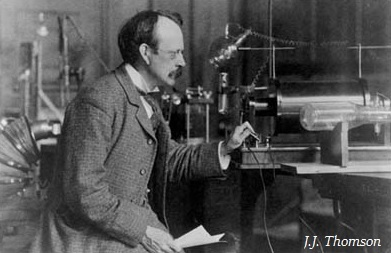• J.J. Thomson was the first person who proposed a model for the structure of an atom.

• In 1886, E. Goldstein discovered the presence of new radiations in a gas discharge and named them canal rays.

• Another positively charged sub-atomic particle was discovered with experiments of canal rays and named it proton.

## Thomson’s Model of Atom

• Thomson proposed that an atom consists of a positively charged sphere and the electrons (negative charge) are embedded in it (as shown in the image given below).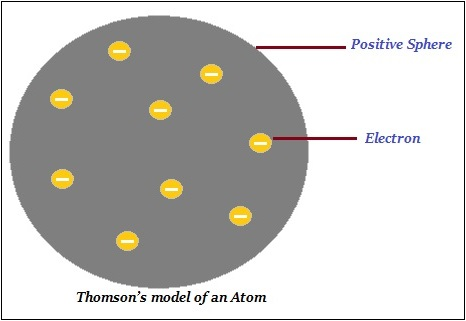• Further, Thomson said that the negative and positive charges are equal in magnitude. Thus, the atom as a whole is electrically neutral.

## Rutherford’s Model of Atom

• E. Rutherford is popular as the ‘Father’ of nuclear physics.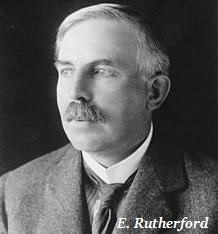• Rutherford is largely known for his work on radioactivity and the discovery of the nucleus of an atom with the gold foil experiment (as shown in the image given below.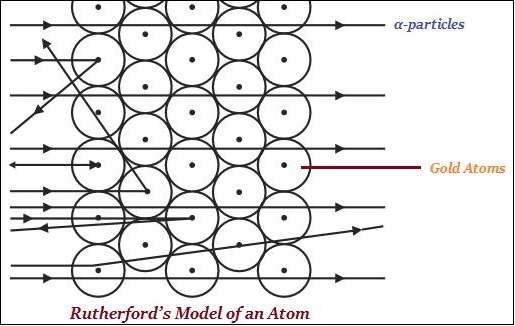• Rutherford said that in an atom, there is a positively charged center known as the nucleus.

• Rutherford said that nearly all the mass of an atom exists in in the nucleus.

• According to Rutherford, the electrons revolve around the nucleus in well-defined orbits.

## Bohr’s Model of Atom

• Neils Bohr further extended Rutherford’s model and improved his drawbacks.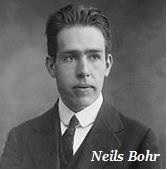• According to Bohr, only certain special orbits known as discrete orbits of electrons, are allowed inside the atom.

• Bohr said that electrons do not radiate energy while revolving in discrete orbits.

• Bohr named orbits or shells as energy levels (as shown in the image given below).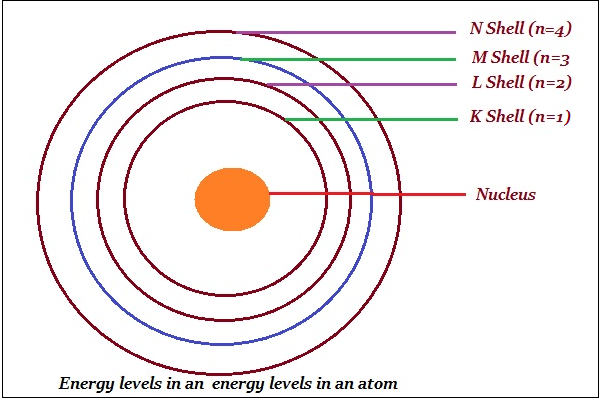• Bohr represented these orbits or shells are by the letters K, L, M, N,… or the numbers, n = 1,2,3,4,….

## Neutron

• In 1932, J. Chadwick discovered a new sub-atomic particle i.e. neutron.

• Neutron has no charge and a mass nearly equal to that of a proton.

• Neutrons are present in the nucleus of all atoms, except hydrogen.

## Electrons Distributed in Different Orbits (Shells)

• The maximum number of electrons that can be present in a shell is given by the formula 2n2.

• ‘n’ is the orbit number or energy level index, i.e. 1, 2, 3,….

• According to the given formula −

• First orbit i.e. K-shell will be = 2 × 12 = 2

• Second orbit i.e. L-shell will be = 2 × 22 = 8

• Third orbit i.e. M-shell will be = 2 × 32 = 18

• Fourth orbit i.e. N-shell will be = 2 × 42 = 32

• Likewise, the maximum number of electrons that can be accommodated in the outermost orbit is 8.

• Electrons are not filled in a given shell, unless the inner shells are filled. It means, the shells are filled in a step-wise manner; starting from inner shell to outer shell.

## Valence

• The electrons, those are present in the outermost shell of an atom, are known as the valence electrons.

• According to Bohr-Bury model, the outermost shell of an atom can have a maximum of 8 electrons.

## Atomic Number

• The total number of protons, present in the nucleus of an atom, is known as atomic number.

• The number of protons of an atom determines the atomic number.

• Atomic number is denoted by ‘Z’.

• Protons and neutrons collectively are known as nucleons.

## Mass Number

• The sum of the total number of protons and neutrons, present in the nucleus of an atom, is known as mass number.

## Isotopes

• The atoms of the same element, having the same atomic number but different mass numbers, is known as isotopes. E.g. Hydrogen atom has three isotopes namely protium, deuterium, and tritium.

• The chemical properties of isotopes of an atom are similar but their physical properties are different.

## Isobars

• Atoms of different elements with different atomic numbers, which have the same mass number, are known as isobars. E.g. calcium’s atomic number is 20and argon’s atomic number is 18; further, the number of electrons in these atoms is different, but the mass number of both these elements is 40.